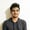7 days of WordPress plugins, themes & templates - for free!* Unlimited asset downloads! Start 7-Day Free Trial

# PHP round, ceil, and floor: Which One to Use?Read Time: 4 mins

A lot of programs, both big and small, require us to deal with numbers. There are two ways of defining numbers in PHP. They can either be floats or integers. We can also use strings to represent very large numbers. You can learn more about these data types and converting between them in this tutorial on integers, floats, and number strings in PHP.

Here, our focus will be on some functions related to integers and floats. We will be discussing floor(), ceil(), and round() in this tutorial.

## Definitions

Let's start with the purpose of all the functions.

1. floor(): This function rounds floats down to the preceding whole number. For example, 5.2 becomes 5, and 8.9 becomes 8.
2. ceil(): This function rounds floats up to whole numbers. For example, 5.2 becomes 6, and 8.9 becomes 9.
3. round(): This function returns a float that has been rounded up or down and approximated to the specified precision. If you don't specify any precision, it will round to the nearest whole number: 5.2 becomes 5, and 8.9 becomes 9.

Remember that the return value of these functions will be integers mathematically speaking, but they would still be a floating point data type.

## Which One Should You Use?

All the functions are useful in specific situations that we will cover below:

### PHP floor() vs. PHP ceil()

The floor() and ceil() functions are useful when you're dealing with situations which only require the use of whole numbers. Consider the following two examples:

Say you have to write a program which determines the number of containers that would be needed to ship some eggs to market. We are bound by two restrictions here. First, containers cannot be fractions. Second, we need to ship all the eggs. This means that we will need a whole container, even if it is not filled up entirely. So we should use ceil().

Consider another situation where you have to determine how many people can be fully fed with the available food. In this case, you only have to invite people if you are certain they can be fed with the available food without ordering anything extra. We will have to ignore the leftover food which cannot feed an adult. So we should use floor().

### PHP round()

The PHP round() function is different from floor() and ceil() because it takes us to the nearest integer (when no precision is specified) instead of always rounding up or down. It takes three parameters:

1. The number you want to round.
2. The precision for rounding the number. The default value is zero, which means no digits after the decimal.
3. The mode for rounding numbers that are halfway, like 3.5, 2.5, and -4.5.

This function is usually used to simplify numbers in everyday situations where we don't need full precision. Scientifically, we can also use round() to get rid of additional spurious precision added by calculations over the precision of data used in those calculations.

In short, for everyday life, using round() is helpful in situations where the exact value of a number is not important and we need an approximation that's easy to remember.

## Final Thoughts

In this tutorial, we have briefly discussed how to use floor(), ceil(), and round(). The floor() function is useful when you always want to get the next lowest integer, and ceil() is useful when you want to get the next highest integer. The round() function is used to round to the closest number (integer or otherwise) with the specified precision.